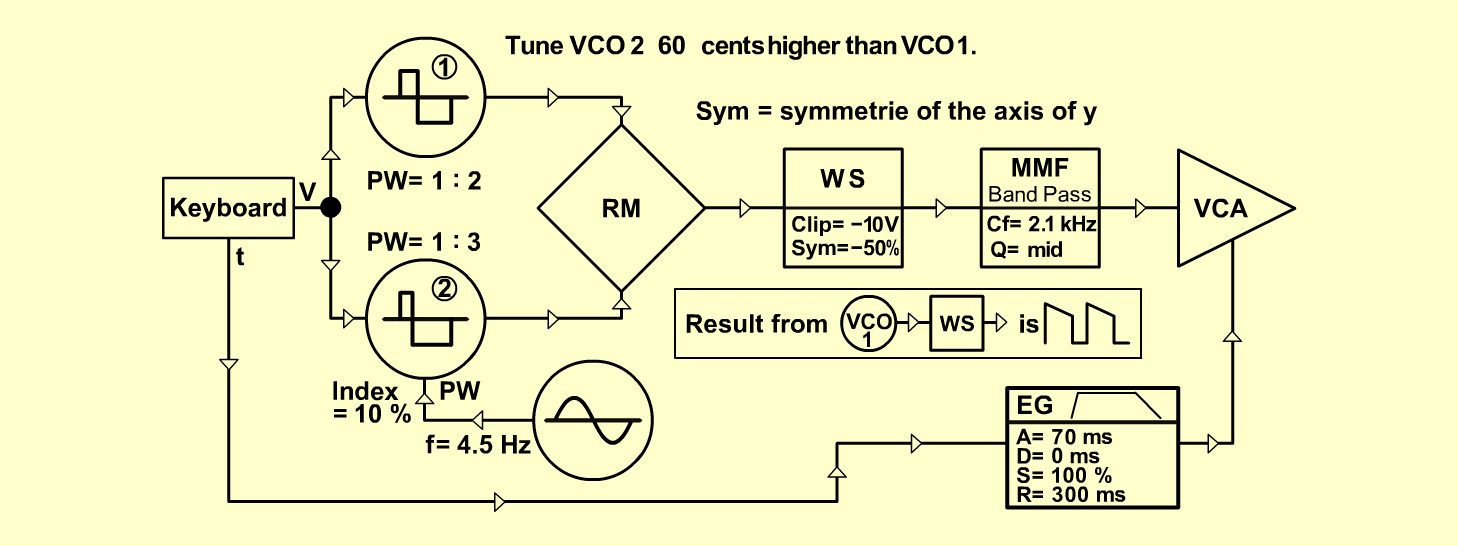HarmonicaInstrumentsD o e p f e r A - 100 Connections: Settings: A-110/1 (Pulse)  <=>  A-114 (X In) A-110/1 (Saw)  <=>  A-110/2 (Sync) A-110/2 (Pulse)  <=>  A-114 (Y In) A-114 (Out)  <=>  A-116 (Audio In) A-116 (Audio Out)  <=>  A-121 (Audio In) A-121 (Band)  <=>  A-131 (Audio In) A-145 (Sine)  <=>  A-110/2 (PW CV 2) A-140 (Output)  <=>  A-131 (CV 1) A-131 (Audio Out)  <=> Amplifier A-110/1 ( PW = 4) A-110/2 ( PW = 3, PW CV 2 = 1) A-116 (Lev = 10, Clip Level = 0, Sym = 2.5) A-121 (Audio Level = 10, Freq = 7, Res = 5) A-145 (Frq = 10, Range = L) A-140 (A = 3, D = 7, S = 6, R = 6, Range = M) A-131 (Gain = 0, Audio In 1 = 10,  Audio Out = 10) Notes: Tune A-110/2  60  Cents higher than A-110/1. Josef MuellerSound samples Harmonica-Piece Harmonica (784 Hz) Harmonica (1046 Hz)